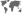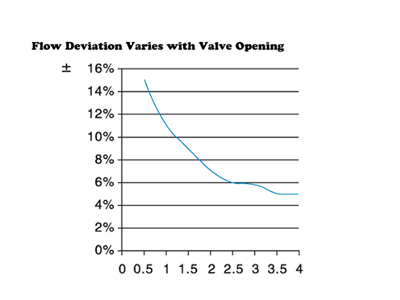﻿ ZECO Valve Test System - ZECO Valve Group
+86-21-5959-9008En
• En

# ZECO Valve Test System

## 1、Valve Flow Characteristic Test

Install the test valve in the corresponding position of the pipeline according to its size, start the frequency converter to make its frequency rise according to the specified rate, the pump speed rises, the pressure difference on both sides of the valve changes accordingly, and get the corresponding flow value at the same time. When the pressure difference between the two sides of the valve reaches its maximum pressure difference or the operating frequency reaches 50HZ, the frequency converter automatically slows down and stops. The data of flow sensor and differential pressure sensor were recorded, and the differential pressure flow characteristic curve was drawn to complete the experiment. FIG. 1 shows the test results of flow characteristic curve of flow balance valve.Figure 1: flow balance valve flow-pressure differential curve

## 2、Valve Opening Characteristic Test

Install the regulator with actuator on the pipeline, adjust the opening of the valve to adjust the flow, and control the pressure difference between the two ends of the valve at 100kPa to obtain the open-flow characteristic curve. See figure 2.Figure 2: Open-flow Curve

## 3、Valve Flow Coefficient, Valve Resistance Coefficient Test

The pressure difference between the two ends of the water valve is controlled at 100kPa, and the flow rate of the valve is measured at this time. The flow coefficient can be obtained by using formula (1), and the resistance coefficient can be calculated by formula (2), where V is the flow rate of the valve pipeline, calculated by formula (3), and D is the nominal diameter of the valve. After the above procedure enters the test option, the report is automatically generated by the computer.

## 4、Pressure Regulating Test of Pressure Reducing Valve and Pressure Differential Valve

The test bench can be used to test the output pressure and flow and input pressure curve of the pressure reducing valve;The pressure difference between the two ends of the differential pressure control valve and the variation curve of flow and input pressure can be measured by using the experimental table.

Related News
Related Water Valves